• +91 9971497814
• info@interviewmaterial.com

# RD Chapter 11- Co-ordinate Geometry Ex-11.1 Interview Questions Answers

### Related Subjects

Question 1 : In a ∆ABC, if ∠A = 55°, ∠B = 40°, find ∠C.

∵ Sum of three angles of a triangle is 180°
∴ In ∆ABC, ∠A = 55°, ∠B = 40°
But ∠A + ∠B + ∠C = 180° (Sum of angles of a triangle)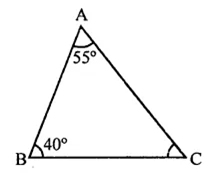⇒ 55° + 40° + ∠C = 180°
⇒ 95° + ∠C = 180°
∴ ∠C= 180° -95° = 85°

Question 2 : If the angles of a triangle are in the ratio 1:2:3, determine three angles.

Ratio in three angles of a triangle =1:2:3
Let first angle = x
Then second angle = 2x
and third angle = 3x
∴ x + 2x + 3x = 180° (Sum of angles of a triangle)
⇒6x = 180°
⇒x =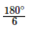= 30°
∴ First angle = x = 30°
Second angle = 2x = 2 x 30° = 60°
and third angle = 3x = 3 x 30° = 90°
∴ Angles are 30°, 60°, 90°

Question 3 : The angles of a triangle are (x – 40)°, (x – 20)° and (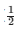x – 10)°. Find the value of x.

Sum of three angles of a triangle = 180°
(x –40)° + (x – 20)° + (x-10)0 = 180°

x –40° + x – 20° +x – 10° = 180°

x +x+x – 70° = 180°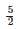x = 180° + 70° = 250°

x=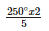= 100°

x =100°

Question 4 : Two angles of a triangle are equal and the third angle is greater than each of those angles by 30°. Determine all the angles of the triangle.

Let each of the two equal angles = x
Then third angle = x + 30°
But sum of the three angles of a triangle is 180°
∴ x + x + x + 30° = 180°
⇒ 3x + 30° = 180°
⇒3x = 150° ⇒x =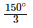= 50°
∴ Each equal angle = 50°
and third angle = 50° + 30° = 80°
∴ Angles are 50°, 50° and 80°

Question 5 : If one angle of a triangle is equal to the sum of the other two, show that the triangle is a right triangle.

In the triangle ABC,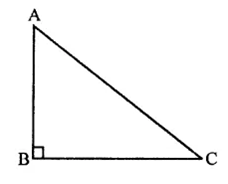∠B = ∠A + ∠C
But ∠A + ∠B + ∠C = 180°
⇒∠B + ∠A + ∠C = 180°
⇒∠B + ∠B = 180°
⇒2∠B = 180°
∴ ∠B =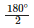= 90°
∵ One angle of the triangle is 90°
∴ ∆ABC is a right triangle.

Question 6 :
Can a triangle have:
(i) Two right angles?
(ii) Two obtuse angles?
(iii) Two acute angles?
(iv) All angles more than 60°?
(v) All angles less than 60°?
(vi) All angles equal to 60°?

(i) In a triangle, two right-angles cannot be possible. We know that sum of three angles is 180° and if there are two right-angles, then the third angle will be zero which is not possible.
(ii) In a triangle, two obtuse angle cannot be possible. We know that the sum of the three angles of a triangle is 180° and if there are
two obtuse angle, then the third angle will be negative which is not possible.
(iii) In a triangle, two acute angles are possible as sum of three angles of a trianlge is 180°.
(iv) All angles more than 60°, they are also not possible as the sum will be more than 180°.
(v) All angles less than 60°. They are also not possible as the sum will be less than 180°.
(vi) All angles equal to 60°. This is possible as the sum will be 60° x 3 = 180°.

Question 7 : The angles of a triangle are arranged in ascending order of magnitude. If the difference between two consecutive angle is 10°, find the three angles.

Let three angles of a triangle be x°, (x + 10)°, (x + 20)°
But sum of three angles of a triangle is 180°
∴ x + (x+ 10)° + (x + 20) = 180°
⇒ x + x+10°+ x + 20 = 180°
⇒ 3x + 30° = 180°
⇒ 3x = 180° – 30° = 150°
∴ x == 50°
∴ Angle are 50°, 50 + 10, 50 + 20
i.e. 50°, 60°, 70°

Question 8 : ABC is a triangle is which ∠A = 72°, the internal bisectors of angles B and C meet in O. Find the magnitude of ∠BOC.

In ∆ABC, ∠A = 12° and bisectors of ∠B and ∠C meet at O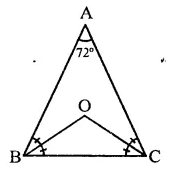Now ∠B + ∠C = 180° – 12° = 108°
∵ OB and OC are the bisectors of ∠B and ∠C respectively
∴ ∠OBC + ∠OCB =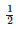(B + C)
=x 108° = 54°
But in ∆OBC,
∴ ∠OBC + ∠OCB + ∠BOC = 180°
⇒ 54° + ∠BOC = 180°
∠BOC = 180°-54°= 126°
OR
According to corollary,
∠BOC = 90°+∠A
= 90+x 72° = 90° + 36° = 126°

Question 9 : The bisectors of base angles of a triangle cannot enclose a right angle in any case.

In right ∆ABC, ∠A is the vertex angle and OB and OC are the bisectors of ∠B and ∠C respectively
To prove : ∠BOC cannot be a right angle
Proof: ∵ OB and OC are the bisectors of ∠B and ∠C respectively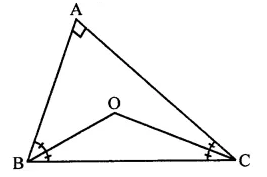∴ ∠BOC = 90° x∠A
Let ∠BOC = 90°, then∠A = O
⇒∠A = O
Which is not possible because the points A, B and C will be on the same line Hence, ∠BOC cannot be a right angle.

Question 10 : If the bisectors of the base angles of a triangle enclose an angle of 135°. Prove that the triangle is a right triangle.

Given : In ∆ABC, OB and OC are the bisectors of ∠B and ∠C and ∠BOC = 135°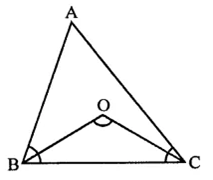To prove : ∆ABC is a right angled triangle
Proof: ∵ Bisectors of base angles ∠B and ∠C of the ∆ABC meet at O
∴ ∠BOC = 90°+∠A
But ∠BOC =135°
∴ 90°+∠A = 135°∠A= 135° -90° = 45°
∴ ∠A = 45° x 2 = 90°
∴ ∆ABC is a right angled triangle

Todays Deals### RD Chapter 11- Co-ordinate Geometry Ex-11.1 Contributorskrishan

Name:
Email:

# Latest News# 9000 interview questions in different categories